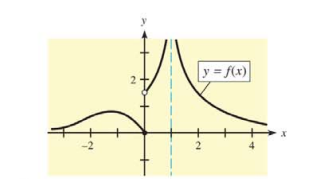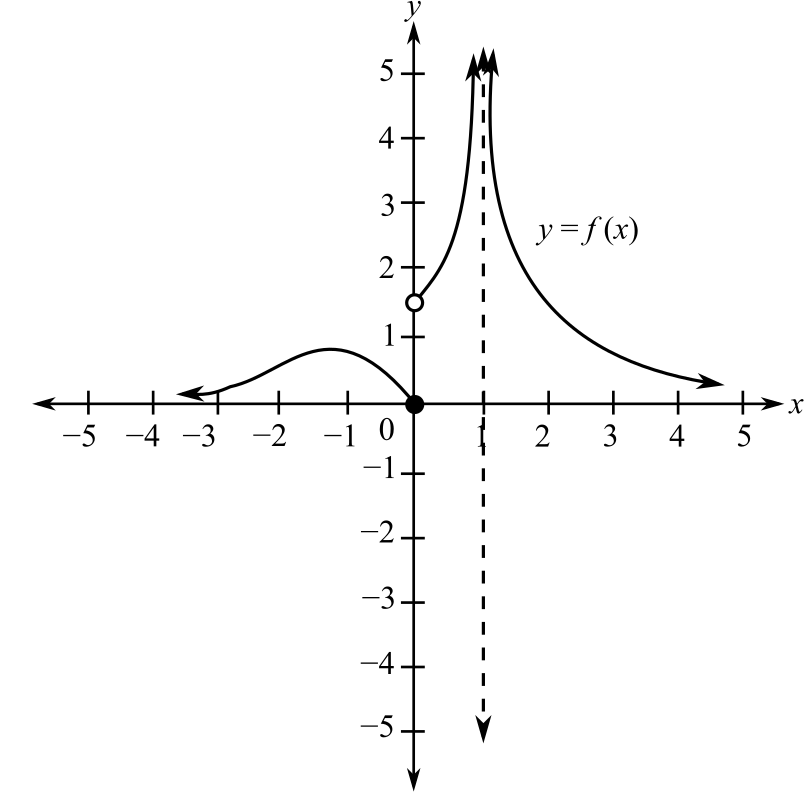Chapter 10, Problem 18RE### Mathematical Applications for the ...

11th Edition
Ronald J. Harshbarger + 1 other
ISBN: 9781305108042

#### Solutions

Chapter
Section### Mathematical Applications for the ...

11th Edition
Ronald J. Harshbarger + 1 other
ISBN: 9781305108042
Textbook Problem

# In Problems 18 and 19, use the graphs to find the following items.(a) vertical asymptotes(b) horizontal asymptotes(c) lim x → ∞ f ( x ) (d) lim x → − ∞ f ( x )(a)

To determine

The vertical asymptote for the provided graph of a function.Explanation

Given Information:

The provided graph is

.

Explanation:

Consider the provided graph,

Recall that a vertical asymptote of a function f(x) is a line x=</

(b)

To determine

The horizontal asymptote for the provided graph of a function.(c)

To determine

The value of limxf(x) for the provided graph of a function.(d)

To determine

The value of limxf(x) for the provided graph of a function.### Still sussing out bartleby?

Check out a sample textbook solution.

See a sample solution

#### The Solution to Your Study Problems

Bartleby provides explanations to thousands of textbook problems written by our experts, many with advanced degrees!

Get Started

#### Find more solutions based on key concepts